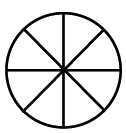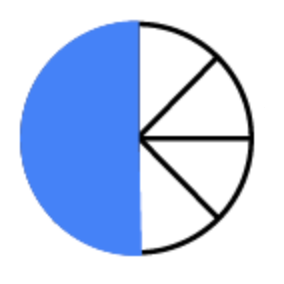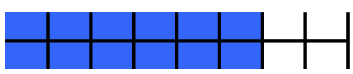### Home > MC1 > Chapter 2 > Lesson 2.1.3 > Problem2-31

2-31.

How many $\frac { 1 } { 8 }$'s are there in each number below?

It may help if you use a Gazinch for this question. If you have not been instructed to do so, you can also draw diagrams for each problem.

1. $\frac { 1 } { 2 }$

The circle below is divided into eighths. Shade in half of the circle.

Then, count the $\frac{1}{8}$’s to answer this question.

There are four  $\frac{1}{8}$’s in $\frac{1}{2}$.1. $\frac { 3 } { 4 }$

Try drawing a picture, like the one below, to help you better understand and solve this problem.1. $\frac { 7 } { 8 }$

Look to the numerator (the top number of the fraction) for help on this problem.

1. $1\frac{1}{2}$

Eight $\frac{1}{8}$'s are in one whole.

Use this information and the information from part (a) to solve this problem.

1. $2$

There are sixteen $\frac{1}{8}$’s in $2$.

1. $3\frac{1}{4}$

Remember it may be helpful to use pictures to solve these problems. Keep up the good work!